# Smoothness, modulus of

(diff) ← Older revision | Latest revision (diff) | Newer revision → (diff)

The modulus of continuity of the derivative of orderof a functiondefined on a Banach space, i.e. the expression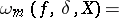where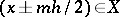. If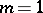, the modulus of smoothness is the ordinary modulus of continuity (cf. Continuity, modulus of) of. Basic properties of the modulus of smoothness (in the caseand the space of continuous functions) are: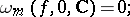does not decrease together with;

if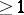is an integer, then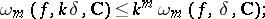for any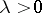,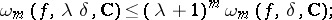if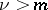, then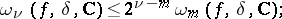if, then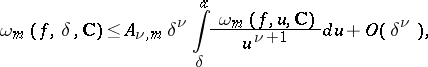where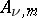andare constants independent of.

Certain problems in the theory of approximation of functions can ultimately be solved only in terms of a modulus of smoothness of order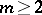. In the theory of approximations of functions an important class is the class of periodic continuous functions with period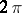and with second-order modulus of smoothness satisfying the condition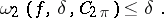The modulus of continuity of such functions satisfies the condition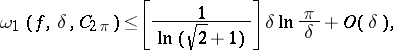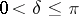, and the constant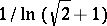cannot be improved .

How to Cite This Entry:
Smoothness, modulus of. Encyclopedia of Mathematics. URL: http://encyclopediaofmath.org/index.php?title=Smoothness,_modulus_of&oldid=12777
This article was adapted from an original article by A.V. Efimov (originator), which appeared in Encyclopedia of Mathematics - ISBN 1402006098. See original article